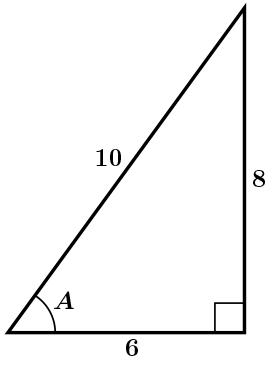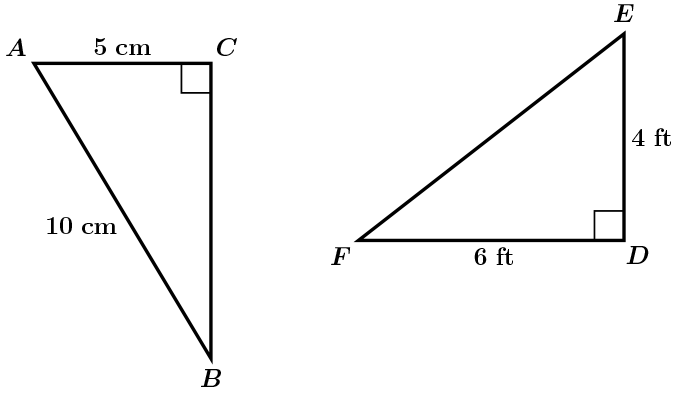# GEOM 2 | Lesson 3 | Practice (Finding Angles)# Finding Angles

Refer back to the concept of arc tan, or inverse tan, to understand the concept of inverse sine and inverse cosine.  Watch (Tangent)

Reminder:  the angle of elevation and angle of depression have the same measure.

Use the following problems to apply the inverse tangent, sine, and cosine functions:

1) Find the angle of elevation to the airplane from the ground:2) Find the measure of angle A.  Which ratio did you use and why?3) Find the measure of angle A and the measure of angle F.